Complimentary 1-hour tutoring consultation

MCAT Content / Sound / Dopplers Effect

### Doppler Effect

Topic: Sound

Doppler’s effect explains the perceived increase (or decrease) in the frequency of sound, light, or other waves as the source and observer move toward (or away from) each other.

The Doppler effect is an alteration in the observed frequency of a sound due to motion of either the source or the observer. What causes the Doppler shift?  The figure below illustrates sound waves emitted by stationary and moving sources in a stationary air mass. Each disturbance spreads out spherically from the point at which the sound is emitted. If the source is stationary, then all of the spheres representing the air compressions in the sound wave are centered on the same point, and the stationary observers on either side hear the same wavelength and frequency as emitted by the source (case a). If the source is moving, the situation is different. Each compression of the air moves out in a sphere from the point at which it was emitted, but the point of emission moves. This moving emission point causes the air compressions to be closer together on one side and farther apart on the other. Thus, the wavelength is shorter in the direction the source is moving (on the right in case b), and longer in the opposite direction (on the left in case b). Finally, if the observers move, as in case (c), the frequency at which they receive the compressions changes. The observer moving toward the source receives them at a higher frequency, and the person moving away from the source receives them at a lower frequency.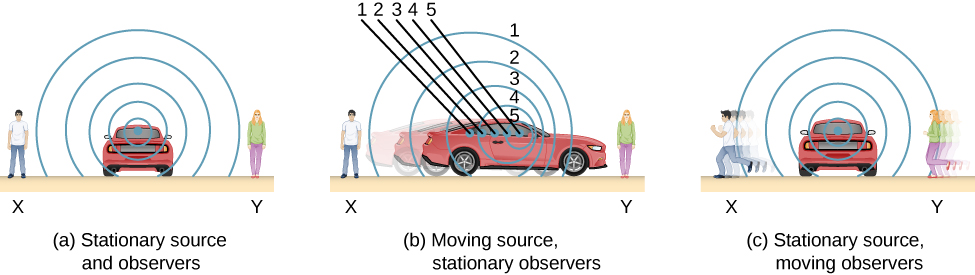We know that wavelength and frequency are related by  where v is the fixed speed of sound. The sound moves in a medium and has the same speed v in that medium whether the source is moving or not. Thus, f multiplied by is a constant. Because the observer on the right in case (b) receives a shorter wavelength, the frequency she receives must be higher. Similarly, the observer on the left receives a longer wavelength, and hence he hears a lower frequency. The same thing happens in case (c). A higher frequency is received by the observer moving toward the source, and a lower frequency is received by an observer moving away from the source. In general, then, relative motion of source and observer toward one another increases the received frequency. Relative motion apart decreases frequency. The greater the relative speed, the greater the effect.

The Doppler effect occurs not only for sound, but for any wave when there is relative motion between the observer and the source. Doppler shifts occur in the frequency of sound, light, and water waves.

Consider two stationary observers located on either side of a stationary source. Each observer hears the same frequency, and that frequency is the frequency produced by the stationary source. Now consider a stationary observer X with a source moving away from the observer with a constant speed . At time , the source sends out a sound wave, indicated in black. This wave moves out at the speed of sound v. The position of the sound wave at each time interval of period  is shown as dotted lines. After one period, the source has moved  and emits a second sound wave, which moves out at the speed of sound. The source continues to move and produce sound waves, as indicated by the circles numbered 3 and 4. Notice that as the waves move out, they remained centered at their respective point of origin.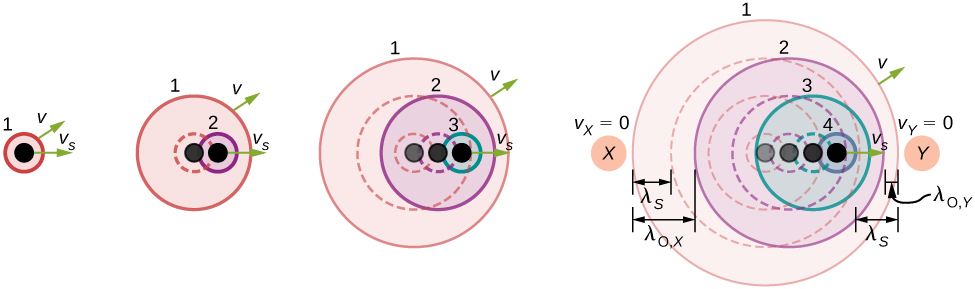Using the fact that the wavelength is equal to the speed times the period, and the period is the inverse of the frequency, we can derive the observed frequency: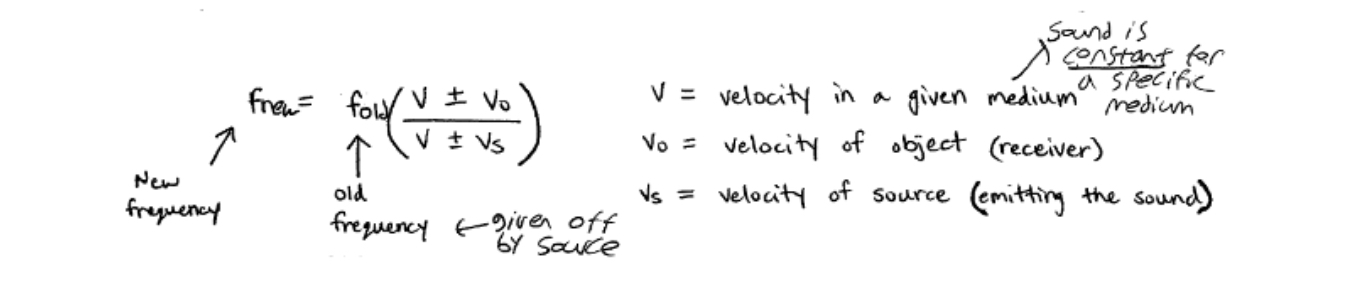As the source moves away from the observer, the observed frequency is lower than the source frequency.

Now consider a source moving at a constant velocity Vs

$,$

moving toward a stationary observer. The wavelength is observed by Y as  Once again, using the fact that the wavelength is equal to the speed times the period, and the period is the inverse of the frequency, we can derive the observed frequency:When a source is moving and the observer is stationary, the observed frequency is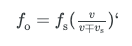where  is the frequency observed by the stationary observer,  is the frequency produced by the moving source, v is the speed of sound, Vs is the constant speed of the source, and the top sign is for the source approaching the observer and the bottom sign is for the source departing from the observer.

What happens if the observer is moving and the source is stationary? If the observer moves toward the stationary source, the observed frequency is higher than the source frequency. If the observer is moving away from the stationary source, the observed frequency is lower than the source frequency. Consider observer X as the observer moves toward a stationary source with a speed . The source emits a tone with a constant frequency  and constant period  The observer hears the first wave emitted by the source. If the observer were stationary, the time for one wavelength of sound to pass should be equal to the period of the source

$.$

Since the observer is moving toward the source, the time for one wavelength to pass is less than Ts and is equal to the observed period  At time

$,$

the observer starts at the beginning of a wavelength and moves toward the second wavelength as the wavelength moves out from the source. The wavelength is equal to the distance the observer traveled plus the distance the sound wave traveled until it is met by the observer:If the observer is moving away from the source, the observed frequency can be found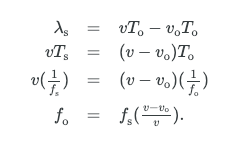The equations for an observer moving toward or away from a stationary source can be combined into one equation: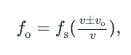where  is the observed frequency,  is the source frequency,  is the speed of sound,  is the speed of the observer, the top sign is for the observer approaching the source and the bottom sign is for the observer departing from the source.

These equations can be further summarized: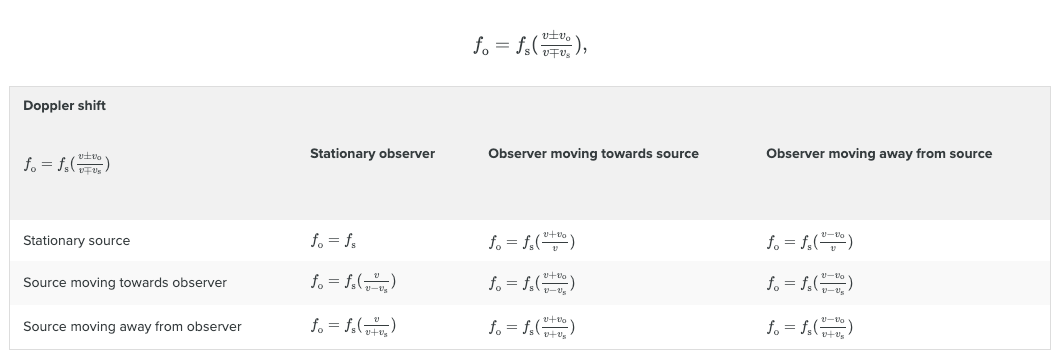where  is the observed frequency,  is the source frequency,  is the speed of sound,  is the speed of the observer,  is the speed of the source, the top sign is for approaching and the bottom sign is for departing.

Practice Questions

How movements influence an ultrasound

MCAT Official Prep (AAMC)

Physics Question Pack Passage 3 Question 13

Physics Question Pack Passage 3 Question 14

Physics Question Pack Passage 3 Question 15

Physics Question Pack Passage 3 Question 16

Physics Question Pack Passage 3 Question 17

Physics Question Pack Passage 3 Question 18

Physics Question Pack Question 40

Physics Question Pack Passage 7 Question 48

Physics Question Pack Question 60

Sample Test C/P Section Passage 10 Question 55

Practice Exam 3 C/P Section Passage 2 Question 6

Key Points

• The Doppler effect is an alteration in the observed frequency of a sound due to motion of either the source or the observer.

• The actual change in frequency is called the Doppler shift.

Key Terms

Frequency: Frequency is the number of occurrences of a repeating event per unit of time. In terms of sound, frequency is perceived as pitch.

Doppler effect: The alteration in the observed frequency of a sound due to motion of either the source or the observer.

Doppler shift: The actual change in frequency due to relative motion of source and observer.

Billing Information